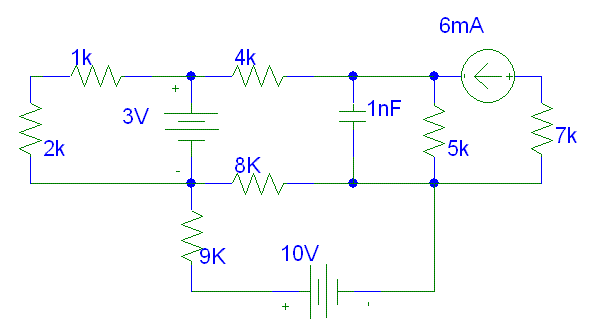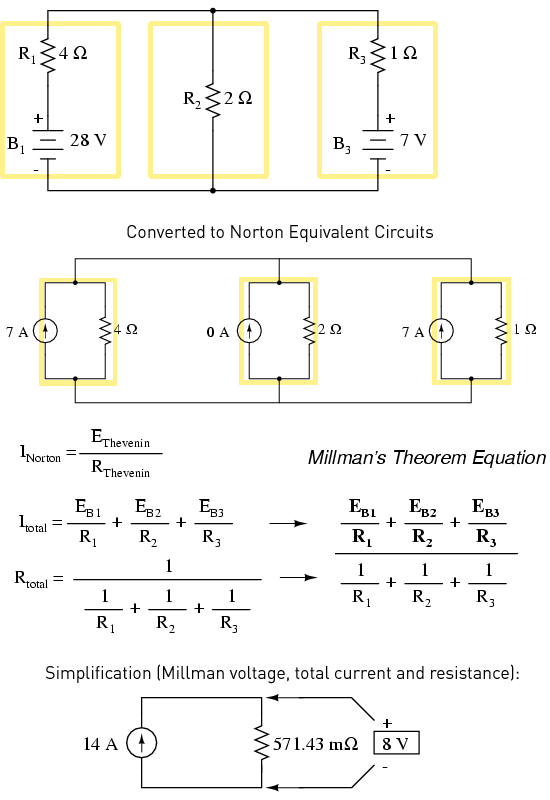# Difference Between Thevenin and Norton

Thevenin and Norton theorems are both related with the electrical systems, but, there are considerable differences between them. The former is applied by using a voltage source while the latter uses a current source.

Thevenin’s theorem was discovered in the late 19th century whereas Norton’s theorem was discovered in the beginning of 20th century. Furthermore, Norton’s theorem is in fact a derivation of Thevenin’s theorem.

Additionally, Thevenin’s theorem makes use of a resistor in an electrical series while Norton’s theorem employs a resistor which is set with the source in a parallel direction.

### Instructions

• 1

Thevenin

It is a theorem related to linear electrical systems. It states that any number of resistors, current and voltage sources can be decreased up to an equal amount of voltage sources and a resistor that is attached with the voltage supply.

It was first discovered in 1853 by a German scientist, Hermann von Helmholtz. It was then rediscovered by Leon Charles Thevenin who was a French telegraph engineer back in 1883. It is considered as one of the most useful theorems in circuit theory and can also be used for AC circuits just by replacing impedance with resistance.

Generally, it is first applied on an open circuit after which the results are used to form and replicate the moves the circuit will make after its path is closed with different kinds of components.

It has great importance as it helps to convert real life components into ideal ones which make the calculations a lot easier.

- Image Courtesy: calvin.edu• 2

Norton

It is a theorem which is also used in linear networking. It states that any quantity of voltage sources, resistors and current sources which have 2 open ends can be easily simplified to create a perfect source of current including a resistor that is attached in a parallel direction to it. Besides, it can also be applied to AC circuits if resistance is replaced by impedance.

It was discovered by two scientists namely Hans Ferdinand Mayer and Edward Lawry Norton in 1926. So, it is also called as Norton-Mayer theorem in some areas of Europe.

This theorem is widely used to simulate different types of electric circuits.

- Image Courtesy: keywon.com•